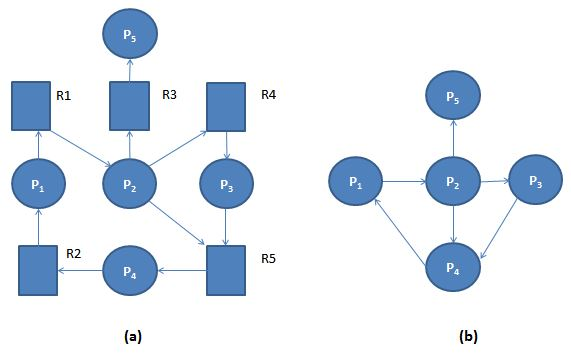Operating Systems

 Allow system to enter deadlock state
 Detection algorithm
 Recovery scheme

### Single Instance of Each Resource Type

 Maintain wait-for graph
» Nodes are processes
» Pi → Pj if Pi is waiting for Pj

 Periodically invoke an algorithm that searches for a cycle in the graph. If there is a cycle, there exists a deadlock

 An algorithm to detect a cycle in a graph requires an order of n2 operations, where n is the number of vertices in the graph

#### Example: (a). 'Resource-Allocation Graph' and Corresponding (b). 'Wait-for Graph'### Several Instances of a Resource Type

Available: A vector of length m indicates the number of available resources of each type.
Allocation: An n x m matrix defines the number of resources of each type currently allocated to each process.
Request: An n x m matrix indicates the current request of each process. If Request [i][j] = k, then process Pi is requesting k more instances of resource type.Rj.

#### Detection Algorithm

```1. Let Work and Finish be vectors of length m and n, respectively Initialize:
(a) Work = Available
(b) For i = 1,2, …, n, if Allocationi ≠ 0, then Finish[i] = false;
otherwise, Finish[i] = true

2. Find an index i such that both:
(a) Finish[i] == false
(b) Requesti ≤ Work
If no such i exists, go to step 4

3. Work = Work + Allocationi Finish[i] = true go to step 2

4. If Finish[i] == false, for some i, 1 ≤ i ≤ n, then the system is in deadlock state. Moreover, if Finish[i] == false, then Pi is deadlocked

```

### Example of Detection Algorithm

 Five processes P0 through P4; three resource types A (7 instances), B (2 instances), and C (6 instances)

 Snapshot at time T0:
AllocationRequestAvailable
A B CA B CA B C
P00 1 00 0 00 0 0
P12 0 02 0 2
P23 0 30 0 0
P32 1 11 0 0
P40 0 20 0 2

 Sequence <P0, P2, P3, P1, P4> will result in Finish[i] = true for all i

 P2 requests an additional instance of type C
Request
A B C
P12 0 0
P23 0 3
P32 1 1
P40 0 2

 State of system?
» Can reclaim resources held by process P0, but insufficient resources to fulfill other processes; requests
» Deadlock exists, consisting of processes P1, P2, P3, and P4

### Detection-Algorithm Usage

 When, and how often, to invoke depends on:
» How often a deadlock is likely to occur?
» How many processes will need to be rolled back?
one for each disjoint cycle

 If detection algorithm is invoked arbitrarily, there may be many cycles in the resource graph and so we would not be able to tell which of the many deadlocked processes “caused” the deadlock.